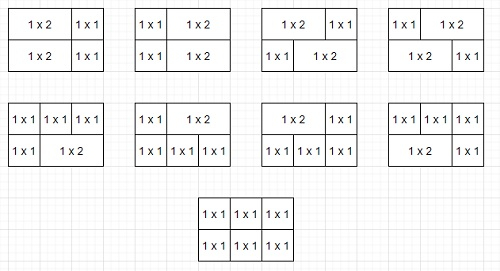# Program to count number of horizontal brick pattern can be made from set of bricks in Python

PythonServer Side ProgrammingProgramming

Suppose we have a list of numbers called bricks and two other values width and height. Each element in bricks[i] represents a brick whose length is bricks[i] units and width is 1 unit. We have to find the number of ways to lay the bricks such that we get full layout of bricks with the given width and height. We can reuse the bricks but can only be laid horizontally.

So, if the input is like bricks = [2, 1] width = 3 height = 2, then the output will be 9 because −To solve this, we will follow these steps −

• w := a list of size same as width and insert 1 at first position, rest are 0
• for i in range 0 to width, do
• if w[i] is non-zero, then
• for each x in bricks, do
• if i + x <= width, then
• w[i + x] := w[i + x] + w[i]
• return w[width]^height

## Example

Let us see the following implementation to get better understanding −

def solve(bricks, width, height):
w =  +  * width
for i in range(width):
if w[i]:
for x in bricks:
if i + x <= width:
w[i + x] += w[i]
return w[width] ** height

bricks = [2, 1]
width = 3
height = 2
print(solve(bricks, width, height))

## Input

[2, 1], 3, 2

## Output

9